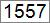Hello Learner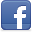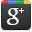Contributor
Stats
 Views : 22191 Comments : 0 Rating : 0
Actions

# Program to generate fibonacci series numbers in .NET

By Ashok Nalam on 30 Dec 2012 | Category: C# | Tagged: number fibonacci
In the this snippet we will generate a series of fibonacci numbers up to no of digits values entered from program.Add Comment   |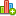Rate this resource

### Introduction

Fibonacci numbers are the numbers in the following sequence of integers.

0, 0, 1, 2, 3, 5, 8, 13, 21, 34, 55….. ( Or )   1,1, 2, 3, 5, 8, 13, 21, 34, 55….

In definition of Fibonacci series the first two numbers are 0 and 1 and then each subsequent number is sum of previous two.So first two numbers we can assign as f0=0 and f1=1. Then f2 value is f0+f1.

F2= f0+f1, f3=f2+f1, f4=f3+f2…………fn=f (n-1) + f (n-2).

The result will be like 0, 0, 1, 2, 3, 5, 8, 13, 21, 34, 55….so on.

In the below program we will generate a sequence of Fibonacci number up to no of value entered.

#### Program:

```class FibonacciSeries
{
public static int Fibonacci(int x)
{
//    F_n = F_{n-1} + F_{n-2} f2=f0+f1
string tempString = string.Empty;
tempString = "The Fibonacci Series for " + x.ToString() + " is:";
var previousValue = -1;
var currentResult = 1;
for (var i = 0; i <= x; ++i)
{
var sum = currentResult + previousValue;
previousValue = currentResult;
currentResult = sum;
tempString += sum + ",";
}
tempString = tempString.Remove(tempString.Length - 1); //to remove , at end of string
Console.WriteLine(tempString);
return currentResult;
}
public static void Main(string[] args)
{
Console.WriteLine("Enter No of digits in Fibonacci series=");
Console.WriteLine("Fibonacci no. = {0}", Fibonacci(MaxSeriesVal));
}
}```

#### Output: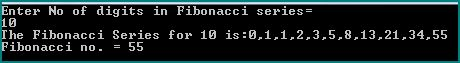Add CommentRate this resource
 Name Email WebSite